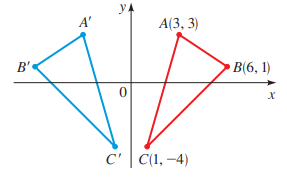# The new coordinates of the point ( 3 , 7 ) when the y -axis acts a mirror and reflect each point to the right of it into a point left of it.### Precalculus: Mathematics for Calcu...

6th Edition
Stewart + 5 others
Publisher: Cengage Learning
ISBN: 9780840068071### Precalculus: Mathematics for Calcu...

6th Edition
Stewart + 5 others
Publisher: Cengage Learning
ISBN: 9780840068071

#### Solutions

Chapter 1.8, Problem 117E

(a)

To determine

## To calculate: The new coordinates of the point (3,7) when the y -axis acts a mirror and reflect each point to the right of it into a point left of it.

Expert Solution

The new coordinates of the point (3,7) are (3,7) .

### Explanation of Solution

Given information:

In the coordinate plane y -axis acts a mirror and reflect each point to the right of it into a point left of it.

Calculation:

Consider the point (3,7) . It is provided that in the coordinate plane y -axis acts a mirror and reflect each point to the right of it into a point left of it.

From the above translation it clear that x- coordinate of each point becomes negative and y -coordinate remains the same.

So, the point (3,7) is reflected to the point (3,7) .

Thus, the new coordinates of the point (3,7) are (3,7) .

(b)

To determine

### To calculate: The new coordinates of the point (a,b) when the y -axis acts a mirror and reflect each point to the right of it into a point left of it.

Expert Solution

The new coordinates of the point (a,b) are (a,b) .

### Explanation of Solution

Given information:

In the coordinate plane y -axis acts a mirror and reflect each point to the right of it into a point left of it.

Calculation:

Consider the point (a,b) . It is provided that in the coordinate plane y -axis acts a mirror and reflect each point to the right of it into a point left of it.

From the above translation it clear that x- coordinate of each point becomes negative and y -coordinate remains the same.

So, the point (a,b) is reflected to the point (a,b) .

Thus, the new coordinates of the point (a,b) are (a,b) .

(c)

To determine

### To calculate: The old coordinates of the point (−4,−1) when the y -axis acts a mirror and reflect each point to the right of it into a point left of it.

Expert Solution

The old coordinates of the point (4,1) are (4,1) .

### Explanation of Solution

Given information:

In the coordinate plane y -axis acts a mirror and reflect each point to the right of it into a point left of it.

Calculation:

Consider the point (4,1) . It is provided that in the coordinate plane y -axis acts a mirror and reflect each point to the right of it into a point left of it.

From the above translation it clear that x- coordinate of each point becomes negative and y -coordinate remains the same.

So, the point (4,1) was originally at the point (4,1) before the reflection.

Thus, the old coordinates of the point (4,1) are (4,1) .

(d)

To determine

### To calculate: The new coordinates of the points A,B and C when the coordinate plane is reflected.Expert Solution

The new coordinates of the points A,B and C are A'=(3,3),B'=(6,1) and C'=(1,4) .

### Explanation of Solution

Given information:

In the coordinate plane y -axis acts a mirror and reflect each point to the right of it into a point left of it.

Calculation:

Consider the triangle ABC reflected o triangle A'B'C' .It is provided that in the coordinate plane y -axis acts a mirror and reflect each point to the right of it into a point left of it.

From the above translation it clear that x- coordinate of each point becomes negative and y -coordinate remains the same.

So, the point A(3,3) is reflected to the point A'(3,3) .

So, the point B(6,1) is reflected to the point B'(6,1) .

So, the point C(1,4) is reflected to the point C'(1,4) .

Thus, the new coordinates of the points A,B and C are A'=(3,3),B'=(6,1) and C'=(1,4) .

### Have a homework question?

Subscribe to bartleby learn! Ask subject matter experts 30 homework questions each month. Plus, you’ll have access to millions of step-by-step textbook answers!